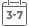# Biochemical Thermodynamics: Applications of Mathematica (Methods of Biochemical Analysis)

• Publish Date: 2006-03-23
• Binding: Hardcover
• Author: Robert A. Alberty
• Sale
• \$18.93
• Regular price \$153.94Navigate the complexities of biochemical thermodynamics with Mathematica(r)

Chemical reactions are studied under the constraints of constant temperature and constant pressure; biochemical reactions are studied under the additional constraints of pH and, perhaps, pMg or free concentrations of other metal ions. As more intensive variables are specified, more thermodynamic properties of a system are defined, and the equations that represent thermodynamic properties as a function of independent variables become more complicated.

This sequel to Robert Alberty's popular Thermodynamics of Biochemical Reactions describes how researchers will find Mathematica(r) a simple and elegant tool, which makes it possible to perform complex calculations that would previously have been impractical. Biochemical Thermodynamics: Applications of Mathematica(r) provides a comprehensive and rigorous treatment of biochemical thermodynamics using Mathematica(r) to practically resolve thermodynamic issues.

Topics covered include:
* Thermodynamics of the dissociation of weak acids
* Apparent equilibrium constants
* Biochemical reactions at specified temperatures and various pHs
* Uses of matrices in biochemical thermodynamics
* Oxidoreductase, transferase, hydrolase, and lyase reactions
* Reactions at 298.15K
* Thermodynamics of the binding of ligands by proteins
* Calorimetry of biochemical reactions

Because Mathematica(r) allows the intermingling of text and calculations, this book has been written in Mathematica(r) and includes a CD-ROM containing the entire book along with macros that help scientists and engineers solve their particular problems.

MORE FROM THIS COLLECTION